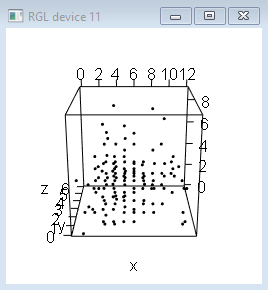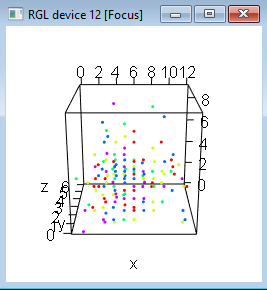# How to create two 3d plots at a time in R?

The rgl package is specifically designed to create real-time interactive 3D plots and we can create two 3d plots using plot3d function of this package. Also, these plots can be viewed in the R console at a single point of time with the help of open3d() function.

## Example

> library(rgl)
> x<-rpois(200,5)
> y<-rpois(200,2)
> z<-rpois(200,3)
> plot3d(x,y,z)

## Output## Example

> open3d()
wgl
12
> plot3d(x,y,z,col=rainbow(5))

## Output